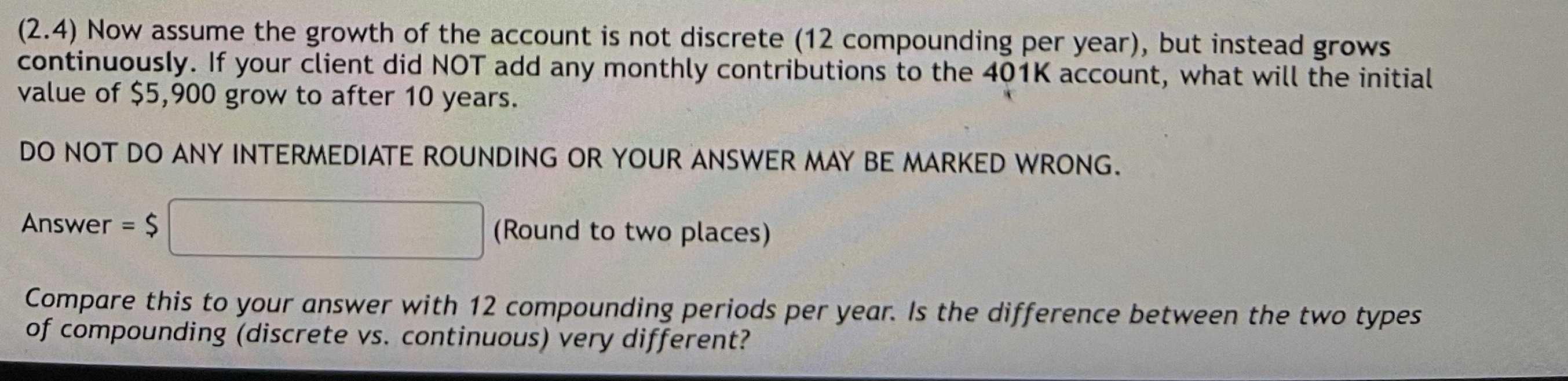### ¿Todavía tienes preguntas de matemáticas?

Pregunte a nuestros tutores expertos
Algebra
Pregunta(2.4) Now assume the growth of the account is not discrete ( $$12$$ compounding per year), but instead grows continuously. If your client did NOT add any monthly contributions to the $$401 K$$ account, what will the initial value of $$\ 5,900$$ grow to after $$10$$ years. DO NOT DO ANY INTERMEDIATE ROUNDING OR YOUR ANSWER MAY BE MARKED WRONG. Answer $$= \ \$$ (Round to two places) Compare this to your answer with $$12$$ compounding periods per year. Is the difference between the two types of compounding (discrete vs. continuous) very different?

$$5900\times (1+ x)^{10\times12}$$